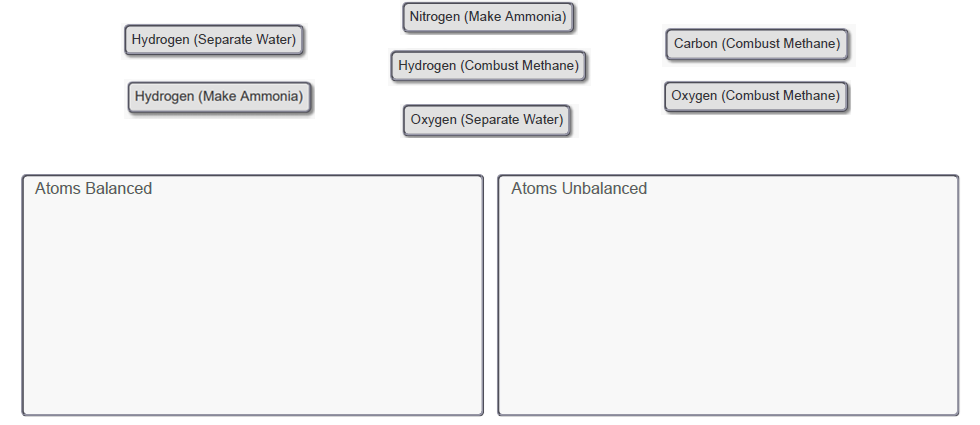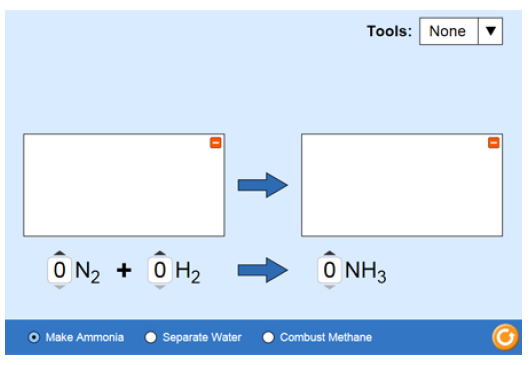Problem: The following unbalanced chemical equations are provided in the PhET simulation:Make Ammonia: N2 + H2 ⟶ NH3Separate Water: H2O ⟶ H2 + O2Combust Methane: CH4 + O2 ⟶ CO2 + H2ODetermine for which elements the specified atoms are balanced or unbalanced in each of these chemical equations when every substance in the reaction is given a coefficient of one (1).Use the PhET simulation to assist you in solving this problem. In the PhET simulation window, click on the Introduction icon at the bottom and select the bar-chart icon from the Tools dropdown menu in the upper right to display how many atoms of each element are present on each side of the chemical equation. To access each chemical equation in PhET, click on the radio button next to each located at the bottom of the screen. For each of the three chemical equations, set the coefficient to "1" for each molecule. Drag the appropriate items to their respective bins.Click on the image to explore the simulation, which tests how molecules are constructed. When you click the simulation link you may be asked whether to run, open, or save the file. Choose to run or open it.When the simulation is open you should select Introduction from the main page. Within the Introduction material, you should see the chemical equation for Make Ammonia with a 0 coefficient set for each molecule. As the coefficient for each molecule is adjusted, that molecule will be displayed on the white rectangle on the correct side of the reaction arrow. There are radio buttons at the bottom to select two other equations as well as a dropdown menu at the top to display the quantity of each atom present in the form of Balance scales or Bar charts. Clicking the Game icon at the bottom brings up a game to test your speed and ability balancing equations.

FREE Expert Solution
100% (118 ratings)
FREE Expert Solution

We are being asked to determine whether the following reactions are balanced

Recall that when balancing chemical equations, we have to make sure that the number of elements on both sides is equal to the lowest whole number coefficients.

100% (118 ratings)
Problem Details

The following unbalanced chemical equations are provided in the PhET simulation:

Make Ammonia: N2 + H2 ⟶ NH3
Separate Water: H2O ⟶ H2 + O2
Combust Methane: CH4 + O2 ⟶ CO2 + H2O

Determine for which elements the specified atoms are balanced or unbalanced in each of these chemical equations when every substance in the reaction is given a coefficient of one (1).

Use the PhET simulation to assist you in solving this problem. In the PhET simulation window, click on the Introduction icon at the bottom and select the bar-chart icon from the Tools dropdown menu in the upper right to display how many atoms of each element are present on each side of the chemical equation. To access each chemical equation in PhET, click on the radio button next to each located at the bottom of the screen. For each of the three chemical equations, set the coefficient to "1" for each molecule.

Drag the appropriate items to their respective bins.Click on the image to explore the simulation, which tests how molecules are constructed. When you click the simulation link you may be asked whether to run, open, or save the file. Choose to run or open it.When the simulation is open you should select Introduction from the main page. Within the Introduction material, you should see the chemical equation for Make Ammonia with a 0 coefficient set for each molecule. As the coefficient for each molecule is adjusted, that molecule will be displayed on the white rectangle on the correct side of the reaction arrow. There are radio buttons at the bottom to select two other equations as well as a dropdown menu at the top to display the quantity of each atom present in the form of Balance scales or Bar charts. Clicking the Game icon at the bottom brings up a game to test your speed and ability balancing equations.